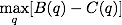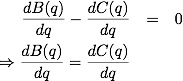## Sunday, January 31, 2010

### Economics

In a post about Ten Principles of Economics, Harvard Professor Greg Mankiw writes a little acrostic device about economics. In addition to things like "Everything has a cost. There is no free lunch. There is always a trade-off." for the "E" in economics, I found this one caught my eye: "One More. Rational people make decisions on the basis of the cost of one more unit (of consumption, of investment, of labor hour, etc.)."

My first thought was, is this really true? Perhaps a real economist will correct me, but I disagree with this statement. From my limited economics knowledge, a rational person makes a decision to maximize the net benefit resulting from that decision, given the information that the person has. This is maximizing the benefits minus the costs, orWhere B(q) is the benefit and C(q) is the cost, and q is a label for the different possible decisions, usually representing the quantity of a particular good that is purchased. To find the maximum (or minimum) we take the derivative and set it to zero:The decision on the "basis of the cost of one more unit" is referring to dC(q)/dq, or the marginal cost. When the marginal cost equals the marginal benefit, then we are at an optimum (which could be a minimum of the net benefit!).

What I disagree with is that I don't think that people make a decision based on dC(q)/dq, but rather that they make decisions on B(q)-C(q). You might say, "But, hey, those are the same thing!". The distinction I think is found in the correspondence between a model and the real-world. Quantities like the cost of a good, or the benefit of the good, are directly observable and measurable. The marginals, or derivatives, of those same quantities are not directly observable but are inferred from the values of the non-marginal quantities at various values of q.

To make an analogy with physics, think of position and speed. Speed is change of position with time, or the "marginal position" if you will. You measure speed by taking different positions and different times of some object, and calculating speed. You don't measure speed directly. The analogy breaks a bit, because there are ways of inferring speed without position, using the doppler effect, which is how we can measure the speeds of very distant objects without knowing their position.

The question I have is, "Is there a similar way to directly measure the marginal cost, without first knowing the cost of the good?" I don't think that this is possible. First, I think it quite likely that there are cases where B(q)-C(q) is not differentiable, so it makes no sense to think of "just one more" but you can still maximize the quantity. The differentiation is a simplification, a model. Second, the equality of dC(q)/dq to dB(q)/dq occurs at both a minimum and a maximum, and needs to be confirmed if it is truly a maximum by going back to the values of B(q) and C(q). Thus knowledge of "one more" is incomplete. Finally, as stated above, I don't think that the marginals are directly observable, whereas the direct quantities are.

Hopefully an economist will comment and correct, or confirm, what I am saying!